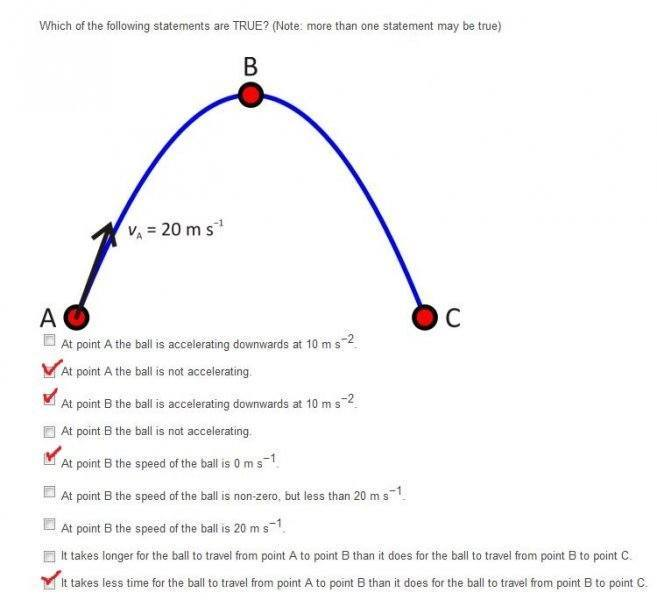# Projectile (Which Statements are true?)

Hi everyone, , I just want to make sure if I have done the statements right. Thank you. :)jedishrfu
Mentor
At point A is the ball being affected by gravity?

Why do you think from A to B takes less time than from B to C or vice versa?

I've counted that one of your checks is correct so far, and that you are missing two others, for a total of three checked boxes. Other members, feel free to correct me if I am wrong.

1. If the ball at A is not accelerating, when does the acceleration begin? What causes the acceleration, and why would it not be constant?
2. While at the peak of a parabolic arc the ball may have a y velocity of 0, does it have no x velocity? If it were to have a velocity in the x direction, would this be greater than, less than, or equal to the initial velocity of the ball at A?
3. Why would the ball travel faster on one part of the arc than another, provided both are equal and symmetrical?

haruspex
Homework Helper
Gold Member
2020 Award
I've counted that one of your checks is correct so far, and that you are missing two others, for a total of three checked boxes. Other members, feel free to correct me if I am wrong.
I concur.
Smart-Bunny, it isn't entirely clear from the diagram, but I would treat the trajectory shown as being merely part of a longer one. So, apart form being equidistant from B, there's nothing special about the points A and C.

Thanks guys!! At point A the ball accelerating downwards 10 m/s2, At point B the ball accelerating downwards 10 m/s2 and at point B the speed is non-zero but less than 20 m/s :)

haruspex